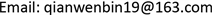1. 静态吸附经验模型的研究进展

d x d t = k ( X − x ) (1)

d x d t = k ( X − x ) (2)

ln ( X X − x ) = k t (3)

x = X ( 1 − e − k t ) (4)

Lagergren一阶动力学表达式很早就被用于预测水溶液中金属离子、染料、有机污染物等的吸附过程。其涵盖的吸附剂种类包括了活性炭  ，生物质材料   ，农业副产物如香蕉皮  等以及无机吸附剂如飞尘  、含氧化锡(IV)水凝胶的聚丙烯酰胺  。描述吸附动力学行为的模型还有扩散模型  、动边界模型  、Stefan-Maxwell模型  等。运用动力学模型对静态吸附实验数据条件下树脂吸附目标物的动力学数据进行系统研究，可得到相关动力学参数如反应速率常数。

The evolution of second kinetic expression and its existing form

Sobkowsk和Czerwinski 1974d θ d t = k ( 1 − θ ) n银电极吸附二氧化碳
Ritchie 1977d θ d t = α ( 1 − θ ) n气体分子的吸附
Blanchard等 1984− d n d t = K ( n 0 − n ) 2金属与铵离子间的离子交换
Ho 1995d q t d t = k ( q e − q t ) 2泥炭吸附二价金属离子

θ为被吸附气体分子所占的吸附位点的分率；n是一个气体分子被吸附所占的吸附位点数；α或k是一个常数或速率常数。Blanchard等式中n是某时刻金属离子被交换的数目或被释放的铵离子数目；n0是指吸附剂平衡交换容量；K是速率常数。Ho等式中k是吸附速率常数；qe是二价金属离子在平衡时的吸附量；qt是二价金属离子吸附剂表面t时刻的吸附量。

2. 动态色谱柱模型

3. 动态色谱柱模型的分类

1) 对流：在色谱柱内，流动相携带着吸附质和溶剂不断沿轴向下出口方向推移，造成这一现象产生的最重要的因素就是对流产生的前进速度。

2) 扩散：是指吸附质在流动相或吸附剂内由于分子扩散产生的轴向位移大小。

3) 传质：也称液膜传质，是指从主体溶液到吸附剂颗粒表面的传质过程。

4) 孔扩散：指的是在吸附剂内孔道的扩散过程。

5) 吸附平衡或吸附速率过程。

GRM模型中吸附质在固相中扩散系数是一个不容易测得的参数，涉及到液相到固相的传质过程。固相扩散系数，也称有效扩散系数，它表示分子在空隙密集的树脂介质中的扩散能力大小。常见分子在水溶液中的自由扩散系数可通过化工手册查得，但是一些特殊分子如未知蛋白质、多肽等，只能通过基团贡献法估算其在水溶液中的扩散系数。

4. 动态色谱柱模型的建模过程

1) 吸附柱床层是均一的，填充的树脂颗粒也是均一的。

2) 上柱液体的密度和粘度不变。

3) 柱内液体流动时的径向扩散忽略不计。

4) 动态吸附操作在等温条件下进行。

N i n = S ε ( c i | z u − D a x ∂ c i ∂ z | z ) (5)

N o u t = S ε ( c i | z + Δ z u − D a x ∂ c i ∂ z | z + Δ z ) (6)

S Δ z ( ε ∂ c i ∂ t + ( 1 − ε ) ∂ q i ∂ t ) | z ¯ , t (7)

S ε ( c i u − D a x ∂ c i ∂ z ) | z , t − S ε ( c i u − D a x ∂ c i ∂ z ) | z + Δ z , t = S Δ z ( ε ∂ c i ∂ t + ( 1 − ε ) ∂ q i ∂ t ) | z ¯ , t (8)

∂ c i ∂ t | z ¯ + 1 − ε ε ∂ q i ∂ t | z ¯ = D a x ( ∂ c i ∂ z ) | z + Δ z , t − D a x ( ∂ c i ∂ t ) | z , t Δ z − u c i | z + Δ z , t − u c i | z , t Δ z (9)

u ∂ c i ∂ z + ∂ c i ∂ t + 1 − ε ε ∂ q i ∂ t = D a x ∂ 2 c i ∂ z 2 (10)

∂ c i , p ∂ t = D i , p 1 r 2 ∂ ∂ r ( r 2 ∂ c i , p ∂ r ) + 1 − ε i ε i k f ( c i − c i , p | r = R p ) (11)

5. 结论与展望